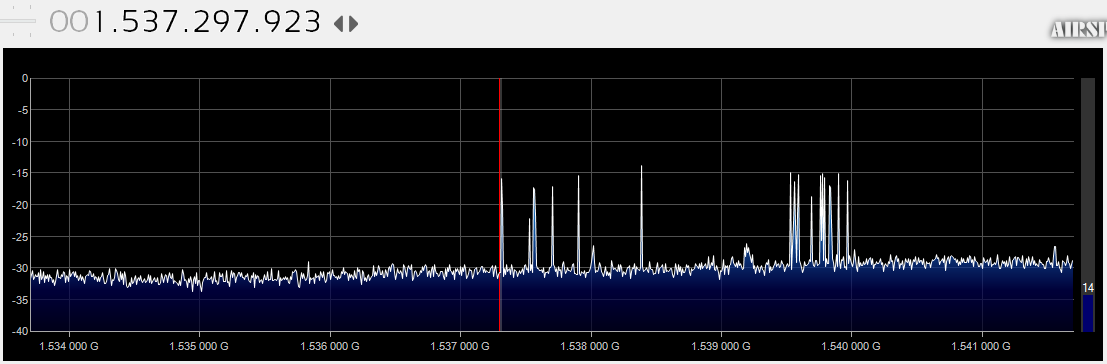This describes a possible multi decoder solution based on: http://aaronscher.com/wireless_com_SDR/RTL_SDR_AM_spectrum_demod.html• Identify the frequencies we want to capture. Our LES’ signal has a 4 kHz frequency band and we aim to capture more than one of these frequency bands at the same time. If say we set the SDR# sample rate to 8 MHz samples/second, we can get several LES at the same time.
• We identify the LES stations and their frequencies.
• We set an SDR# hook into the full IQ bandwidth for the data. The parameters we need at this point are the bandwidth (8 MHz) and the center frequency of the captured data, say 1,538,167,000 Hz.

Q. What is the format of the IQ data?

• If we would now FFT-plot the data samples received, the center frequency of the captured data (1,538,167,000 Hz) would be at 0 Hz in the plot, and the principal alias frequency range will go from -4 MHz to +4 MHz (1,534,167,000 – 1,542,167,000).

For each LES we could then:

• Frequency down shift and frequency up shift our signal multiplying with a complex exponential and we can get any frequency band we want to, pending it is contained within the baseband.
• Say we center our respective LES at 2000 Hz based on the one we want processing.
• The next step is to apply a LPF for our 4000 Hz band. And then decimate the signal to our needed 48000 SR. The LPF will guard against aliasing.
• Decode the 48000 SR signal in the 4 kHz bandwidth.